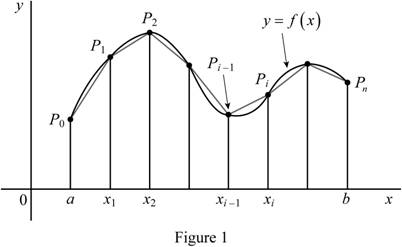Chapter 8, Problem 1RCC

Chapter
Section
Textbook Problem

(a) How is the length of a curve defined? (b) Write an expression for the length of a smooth curve given by y = f(x), a ≤ x ≤ b. (c) What if x is given as a function of y?

(a)

To determine

To Define: The length of the curve.

Explanation

Draw the Figure 1 using the procedure as follows:

• Draw a curve C for equation y=f(x) .
• Here,f is a continuous function and axb .
• Divide the curve as a polygonal curve with n number of subintervals at the interval [a,b] .
• The endpoint is a=x0<x1<x2<...<xn=b . The equal width of subinterval as Δx .
• The point Pi(xi,yi) lies on the curve for yi=f(xi) and the vertices of polygon P0,P1,.....Pn

Show the curve as in Figure 1.Refer Figure 1,

If the limit exists, the length of the curve C with equation y=f(x) , for axb as the limit of the lengths of these inscribed polygons.

L=limni=1n|Pi1Pi|

Thus, the length of the curve is defined.

(b)

To determine

To Express: The length of a smooth curve given by y=f(x),axb .

The expression for the length of a smooth curve is L=ab1+[f(x)]2dx

Explanation

Consider the curve is a straight line between the coordinates (x1,y1) and (x2,y2) . Then the length of curve can be expressed by using Pythagorean Theorem.

L=(Δx)2+(Δy)2 (1)

Here, Δx=x2x1 and Δy=y2y1

Suppose the Equation of curve formed by y=f(x) or x=f(x) where axb, then the Equation(1) can be expressed as

L=ab(dx)2+[f(x)dx]2L=ab1+[f(x)]2dx

Thus, the length of a smooth curve given by y=f(x),axb is defined.

(c)

To determine

To Explain: The equation of curve has x as a function of y.

If x as a function of y the expression of length of curve is L=cd1+[g(y)]2dy

Explanation

Consider x=g(y) where cyd , then f(x)=g(y) therefore the length of curve can be expressed as,

L=cd1+[g(y)]2dy

Thus, the equation of curve has x as a function of y is explained.

The Solution to Your Study Problems

Bartleby provides explanations to thousands of textbook problems written by our experts, many with advanced degrees!

Get Started

Find more solutions based on key concepts